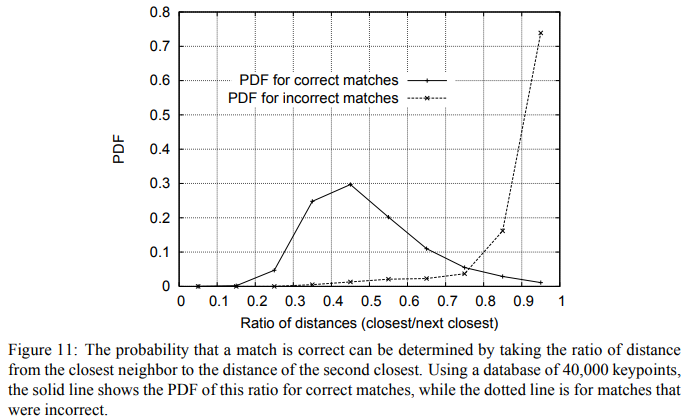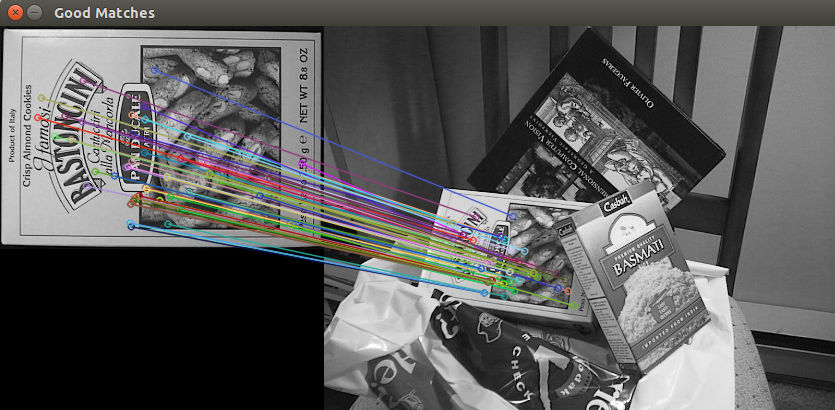OpenCV  3.4.20-dev Open Source Computer Vision
Feature Matching with FLANN

Prev Tutorial: Feature Description

Next Tutorial: Features2D + Homography to find a known object

## Goal

In this tutorial you will learn how to:

Warning
You need the OpenCV contrib modules to be able to use the SURF features (alternatives are ORB, KAZE, ... features).

## Theory

Classical feature descriptors (SIFT, SURF, ...) are usually compared and matched using the Euclidean distance (or L2-norm). Since SIFT and SURF descriptors represent the histogram of oriented gradient (of the Haar wavelet response for SURF) in a neighborhood, alternatives of the Euclidean distance are histogram-based metrics ( $$\chi^{2}$$, Earth Mover’s Distance (EMD), ...).

Arandjelovic et al. proposed in  to extend to the RootSIFT descriptor:

a square root (Hellinger) kernel instead of the standard Euclidean distance to measure the similarity between SIFT descriptors leads to a dramatic performance boost in all stages of the pipeline.

Binary descriptors (ORB, BRISK, ...) are matched using the Hamming distance. This distance is equivalent to count the number of different elements for binary strings (population count after applying a XOR operation):

$d_{hamming} \left ( a,b \right ) = \sum_{i=0}^{n-1} \left ( a_i \oplus b_i \right )$

To filter the matches, Lowe proposed in  to use a distance ratio test to try to eliminate false matches. The distance ratio between the two nearest matches of a considered keypoint is computed and it is a good match when this value is below a threshold. Indeed, this ratio allows helping to discriminate between ambiguous matches (distance ratio between the two nearest neighbors is close to one) and well discriminated matches. The figure below from the SIFT paper illustrates the probability that a match is correct based on the nearest-neighbor distance ratio test.Alternative or additional filterering tests are:

• cross check test (good match $$\left( f_a, f_b \right)$$ if feature $$f_b$$ is the best match for $$f_a$$ in $$I_b$$ and feature $$f_a$$ is the best match for $$f_b$$ in $$I_a$$)
• geometric test (eliminate matches that do not fit to a geometric model, e.g. RANSAC or robust homography for planar objects)

## Result

• Here is the result of the SURF feature matching using the distance ratio test: